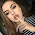## Thursday, November 3, 2016

### High School Math Solutions – Polynomials Calculator, Dividing Polynomials (Long Division)

Last post, we talked dividing polynomials using factoring and splitting up the fraction. In this post, we will talk about another method for dividing polynomials, long division. Long division with polynomials is similar to the basic numerical long division, except we are dividing variables. This is where it gets tricky. I will talk about the steps to dividing polynomials using long division to help make the process easier and go into detail.

Steps for polynomial long division:

1.  Organize each polynomial by higher order
• We want to make sure that each polynomial is written in order of the variable with the highest exponent to the variable with the lowest exponent
• you can skip this step if they are already in high order
2.  Set up in long division form
• The denominator becomes the divisor and the numerator becomes the dividend

3.  Write 0 as the coefficient for missing terms in the dividend
• Since we’ve put in order the terms based on their exponent, we can see which terms are missing (i.e. x^4+x^2 we can see we are missing an x^3 term so we will add that in to its proper spot and make the coefficient 0)
• This will help you with step 6, so you don’t subtract the wrong terms
• You can skip this step if there are no missing terms
4.   Divide the first term of the dividend (numerator) by the first term of the divisor (denominator)
• This is allows us to see what we need to multiply the divisor by to get rid of the first term of the dividend
5.   Multiply the divisor by that term
•  Write the term down on top of line where the term that is getting eliminated is
6.  Subtract this from the dividend
• This gives you a new polynomial to work with
7.  Repeat steps 4-6 until you get a remainder
• When you repeat step 4, move onto the newest first term from step 6
8.  Put the remainder over the divisor to create a fraction and add it to the new polynomial

This may seem a bit confusing, so we will go through two examples step by step to understand better how to solve these problems.

\frac{(x^4+6x^2+2)}{(x^2+5)}

1.  Organize each polynomial by high order
We can skip this step because the polynomials are already in high order
2.  Set up in long division form
3.  Write 0 as the coefficient for missing terms in the dividend

4.  Divide the first term of the dividend (numerator) by the first term of the divisor (denominator)
\frac{x^4}{x^2}= x^2

5.  Multiply the divisor by that term
x^2∙(x^2+5)=x^4+5x^2

6.  Subtract this from the dividend

7.  Repeat step 4-6 until you get a remainder
\frac{x^2}{x^2} =1

8.  Put the remainder over the divisor to create a fraction and add it to the new polynomial
x^2+1+\frac{(-3)}{(x^2+5)}

\frac{(2x^2-18+5x)}{(x+4)}

1.  Organize each polynomial by high order
\frac{(2x^2+5x-18)}{(x+4)}

2.  Set up in long division form

3.  Write 0 as the coefficient for missing terms in the dividend
We can skip this step because there are no missing terms.

4.  Divide the first term of the dividend (numerator) by the first term of the divisor (denominator)
\frac{2x^2}{x}=2x

5.  Multiply the divisor by that term
2x(x+4)=2x^2+8x

6.  Subtract this from the dividend

7.  Repeat steps 4-6 until you get the remainder
\frac{(-3x)}{x}=-3

8.  Put the remainder over the divisor to create a fraction and add it to the new polynomial
2x-3+\frac{(-6)}{(x+4)}

Dividing polynomials using long division is very tricky. It is so easy to skip an exponent, have an algebraic error, and forget a step. This is why practicing this type of problem is so important. The only way to get better at it is to keep practicing it. Check out Symbolab’s Practice for practice problems and quizzes.

Until next time,

Leah.

1.2.video sabung ayam toraja paling seru

3.daftar segera di bandar ayam bangkok terpercaya

4.Mau Banyak Bonus?, Yuk Gabung Disini >> sabung ayam pukul mati

5.situs terpercaya agen sabung ayam

6.7.8.9.mau yang asik ? adu ayam

10.11.This website can live streaming , you can join at my site :
agen judi online terpercaya
Prediksi Bola

Thank you
agenpialaeropa.club
gamesonline.ga
beritasemasaterikini.net

12.Great post i am really impressed with your post thank you for information

คาสิโนออนไลน์ที่น่าเชื่อถือและมีความเป็นมืออาชีพที่สุดในตอนนี้
โปรโมชั่นGclub ของทางทีมงานตอนนี้แจกฟรีโบนัส 50%
เพียงแค่คุณสมัคร สล็อตออนไลน์ กับทางทีมงานของเราเพียงเท่านั้น
ร่วมมาเป็นส่วนหนึ่งกับเว็บไซต์คาสิโนออนไลน์ของเราได้เลยค่ะ
สมัครสล็อตออนไลน์ >>> Goldenslot
สนใจร่วมสนุกกับ คาสิโนออนไลน์ คลิ๊กได้เลย
มีทั้งคาสิโนออนไลน์ หวยออนไลน์ ฟุตบอลออนไลน์ สล็อตออนไลน์ และอื่นๆอีกมากมาย

13.14.15.Hello guys! It is a very interesting post! Practically every time I had been using an essay writing service while studying at high school. One of my favourite French teachers asked me to write a school letter. Mostly, I hated these types of work, so I prefered to pay money for this. And now I use pretty awesome MasterPapers.com website that helps me a lot with all of my school assignments!

16.Nikmati Promo Natal 2019 Dan Semangat Tahun Baru 2020 Bersama kami di BOLAVITA dengan berbagai hadiah yg super menarik dan pastinya cukup menggiurkan untuk anda...

Untuk Infomasi Lebih Lanjut Bisa Hub kami Di :
Wechat : bolavita
Line : cs_bolavita
WA : 0812-2222-995

Ayo segera daftar dan Menangkan Hadiah nya :)

17.Hello guys! It is a very interesting post! Practically every time I had been using an essay writing service while studying at high school. One of my favourite French teachers asked me to write a school letter. Mostly, I hated these types of work, so I prefered to pay money for this.
Vdcasino
Vdcasino
Vdcasino
Ngsbahis
Ngsbahis
Ngsbahis
Betcup
Betcup
Betcup

18.Selam beyler! Çok ilginç bir yazı! Neredeyse her seferinde lisede okurken bir makale yazma servisi kullanıyordum. En sevdiğim Fransız öğretmenlerimden biri benden bir okul mektubu yazmamı istedi. Çoğunlukla bu tür çalışmalardan nefret ettim, bu yüzden bunun için para ödemeyi tercih ettim.
Venüsbet
Klasbahis
Bahsegel
Bahsegel
Supertotobet